# Interior Orientation

## Producer Field Guide

HGD_Product
Producer Field Guide
HGD_Portfolio_Suite
Producer

Interior orientation defines the internal geometry of a camera or sensor as it existed at the time of data capture. The variables associated with image space are defined during the process of interior orientation. Interior orientation is primarily used to transform the image pixel coordinate system or other image coordinate measurement system to the image space coordinate system.

The figure below illustrates the variables associated with the internal geometry of an image captured from an aerial camera, where o represents the principal point and a represents an image point.

Internal Geometry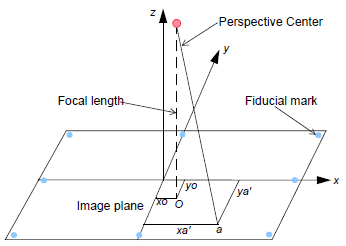The internal geometry of a camera is defined by specifying the following variables:

• Principal point
• Focal length
• Fiducial marks
• Lens distortion

Principal Point and Focal Length

Principal point is mathematically defined as the intersection of the perpendicular line through the perspective center of the image plane. The length from the principal point to the perspective center is called the focal length (Wang, Z., 1990).

The image plane is commonly referred to as the focal plane. For wide-angle aerial cameras, the focal length is approximately 152 mm, or 6 inches. For some digital cameras, the focal length is 28 mm. Prior to conducting photogrammetric projects, the focal length of a metric camera is accurately determined or calibrated in a laboratory environment.

This mathematical definition is the basis of triangulation, but difficult to determine optically. The optical definition of principal point is the image position where the optical axis intersects the image plane. In the laboratory, this is calibrated in two forms: principal point of autocollimation and principal point of symmetry, which can be seen from the camera calibration report. Most applications prefer to use the principal point of symmetry since it can best compensate for the lens distortion.

Fiducial Marks

As stated previously, one of the steps associated with interior orientation involves determining the image position of the principal point for each image in the project. Therefore, the image positions of the fiducial marks are measured on the image, and subsequently compared to the calibrated coordinates of each fiducial mark.

Since the image space coordinate system has not yet been defined for each image, the measured image coordinates of the fiducial marks are referenced to a pixel or file coordinate system. The pixel coordinate system has an x coordinate (column) and a y coordinate (row). The origin of the pixel coordinate system is the upper left corner of the image having a row and column value of 0 and 0, respectively. The following figure illustrates the difference between the pixel coordinate system and the image space coordinate system.

Pixel Coordinate System vs. Image Space Coordinate System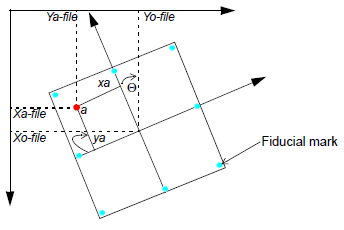Using a two-dimensional affine transformation, the relationship between the pixel coordinate system and the image space coordinate system is defined. The following two-dimensional affine transformation equations can be used to determine the coefficients required to transform pixel coordinate measurements to the image coordinates: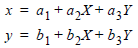The x and y image coordinates associated with the calibrated fiducial marks and the X and Y pixel coordinates of the measured fiducial marks are used to determine six affine transformation coefficients. The resulting six coefficients can then be used to transform each set of row (y) and column (x) pixel coordinates to image coordinates.

The quality of the two-dimensional affine transformation is represented using a root mean square (RMS) error. The RMS error represents the degree of correspondence between the calibrated fiducial mark coordinates and their respective measured image coordinate values. Large RMS errors indicate poor correspondence. This can be attributed to film deformation, poor scanning quality, out-of-date calibration information, or image mismeasurement.

The affine transformation also defines the translation between the origin of the pixel coordinate system and the image coordinate system (xo-file and yo-file). Additionally, the affine transformation takes into consideration rotation of the image coordinate system by considering angle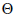(see the "Pixel Coordinate System vs. Image Space Coordinate System" figure above). A scanned image of an aerial photograph is normally rotated due to the scanning procedure.

The degree of variation between the x- and y-axis is referred to as nonorthogonality. The two-dimensional affine transformation also considers the extent of nonorthogonality. The scale difference between the x-axis and the y-axis is also considered using the affine transformation.

Lens Distortion

Lens distortion deteriorates the positional accuracy of image points located on the image plane. Two types of radial lens distortion exist: radial and tangential lens distortion.

Lens distortion occurs when light rays passing through the lens are bent, thereby changing directions and intersecting the image plane at positions deviant from the norm. The figure below illustrates the difference between radial and tangential lens distortion.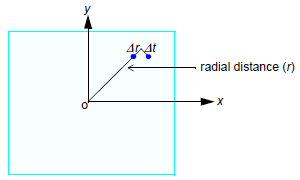Radial lens distortion causes imaged points to be distorted along radial lines from the principal point o.

The effect of radial lens distortion is represented as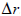. Radial lens distortion is also commonly referred to as symmetric lens distortion.

Tangential lens distortion occurs at right angles to the radial lines from the principal point. The effect of tangential lens distortion is represented as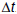. Since tangential lens distortion is much smaller in magnitude than radial lens distortion, it is considered negligible.

The effects of lens distortion are commonly determined in a laboratory during the camera calibration procedure.

The effects of radial lens distortion throughout an image can be approximated using a polynomial. The following polynomial is used to determine coefficients associated with radial lens distortion: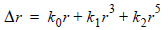represents the radial distortion along a radial distance r from the principal point (Wolf, 1983). In most camera calibration reports, the lens distortion value is provided as a function of radial distance from the principal point or field angle. IMAGINE Photogrammetry Project Manager accommodates radial lens distortion parameters in both scenarios.

Three coefficients (k0, k1, and k2) are computed using statistical techniques. Once the coefficients are computed, each measurement taken on an image is corrected for radial lens distortion.